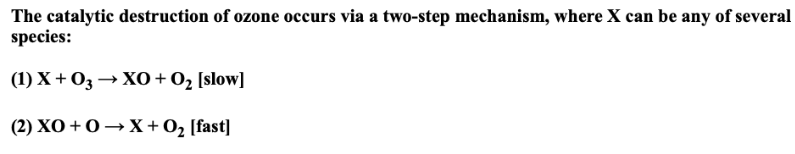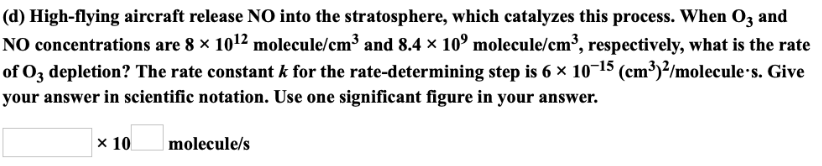# The catalytic destruction of ozone occurs via a two-step mechanism, where X can be any of several species: (1) X + O3 → XO + O2 [slow] (2) XO + O → X + O2 [fast] (d) High-flying aircraft release NO into the stratosphere, which catalyzes this process. When O3 and NO concentrations are 8 x 10^12 molecule/cm^3 and 8.4 x 10^9 molecule/cm^3, respectively, what is the rate of O3 depletion? The rate constant k for the rate-determining step is 6 x 10^-15 (cm^3)^2/molecule.s. Give your answer in scientific notation. Use one significant figure in your answer.